# Improved max flow path augmenting

 Improved max flow path augmenting 1. Augment in "large" increments a) Find max residual path augment along it O (m2 log U) b) Find residual path that is "sufficiently" large. Capacity scaling (Section 7.3) O (m2 log U) Find residual arcs with capacity >  keep a network with only those arcs.   initially =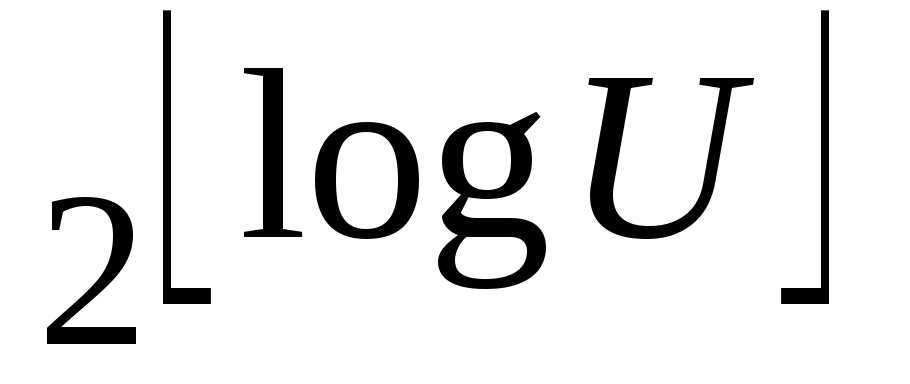 Augment on paths  Update  =  / 2 until  = 1  In each scaling phase ( value) there is a maximum of O(m) augmentations [each augmentation O(m)]  hence O(m2 log U) 2. Augment along shortest path 7.4 (smallest number of arcs) O(n2m) Flavor of shortest path idea in preflow push discussion Preflow Push Algorithms The best preflow push algorithms outperform the best path augmenting in theory and in practice. Push flow along individual arcs, not paths. But, doing this may violate mass balance constraints. Definition: Preflow A preflow is any flow satisfying Flow bound constraints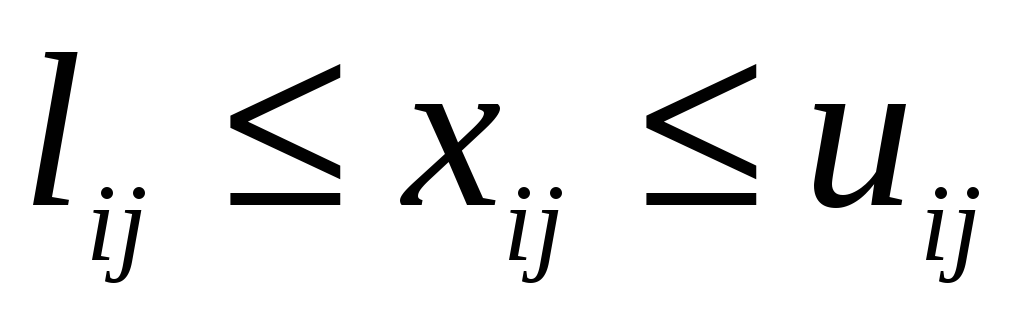2)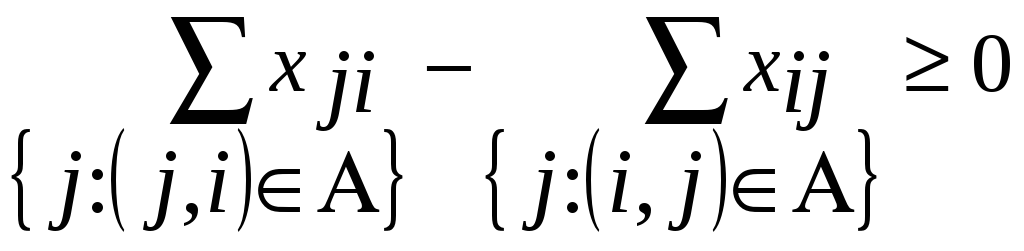for all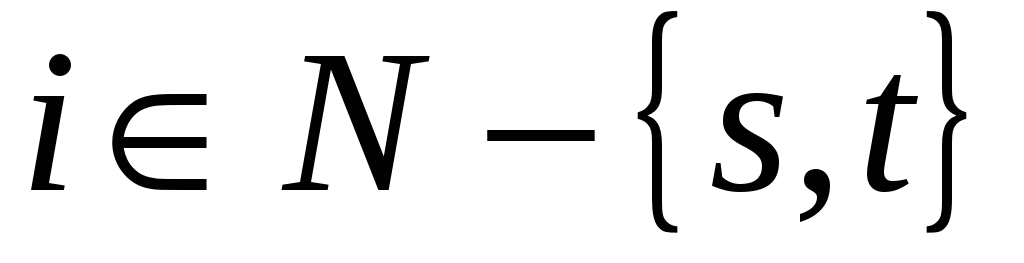Flow entering can exceed flow leaving. Thus, may violate mass balance constraint. The idea is to push flow on arcs, violate mass balance, then restore mass balance (feasibility) for nodes.  Push toward the sink to do this, if we can't push toward the sink then push toward the source. Definition e(i) excess of each node i  N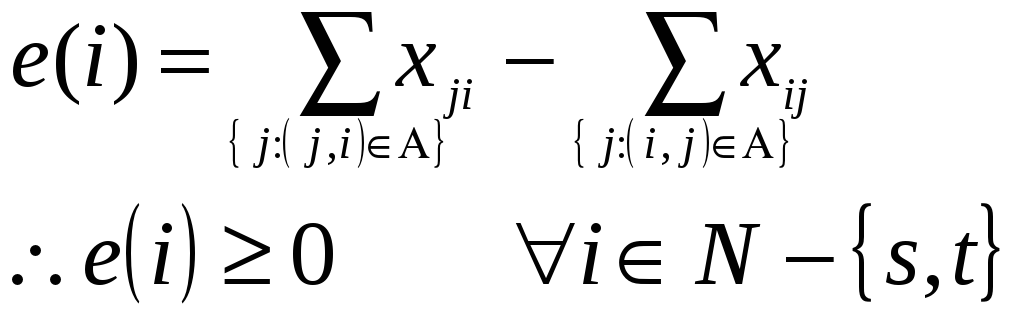Definition An active node i is one with e(i) > 0.  s & t are never active by convention Thus select active nodes, because they are infeasible, and make them feasible. Where to push flow? Closer to the sink Closer means to a node with a shorter distance label Distance Labels What is a distance label? Distance labels, d(i), are set for each node i and have these properties 1. d(t) = 0 2. d(i)  d(j) + 1 for every arc (i, j) in the residual network G(x). Can show Property 7.1 If the distance labels are valid (satisfy 1 & 2 above) then the distance label d(i) is a lower bound on the length of the shortest directed path from node i to node t in the network. Thus property 7.2 is obvious If d(s)  n the residual network has no directed path from s to t. Definition: d(i) is exact if d(i) = the length of the shortest path from i to t in the residual network. Definition: An arc (i, j) in the residual network is admissible if d(i) = d(j) + 1, else it is inadmissible. We only push flow on admissible arcs. Definition: A path from s to t consisting of all admissible arcs is an admissible path. An admissible path is a shortest augmenting path from s to t. Generic Preflow Push Algorithm procedure preprocess; begin x : = 0; compute the exact distance labels d(i); xsj : = usj for each arc (s, j)  A (s); d(s) : = n; end; procedure push/relabel(i); begin if the network contains an admissible arc (i, j) then push  : = min {e(i), rij} units of flow from node i to node j else replace d(i) by min {d(j) + 1 : (i, j)  A(i) and rij > 0}; end; Definition - arc saturated if  = rij algorithm preflow-push; begin preprocess; while the network contains an active node do begin select an active node i; push/relabel (i); end; end; Water Analogy Flexible pipes, joints, d(.) Arcs nodes how high node is off of the ground admissible arc -- downhill Move source up, water flows down. Increase d() until flow goes to sink or source When you are stuck at a node with all uphill flows, increase d() until a downward flow exists Why does the generic preflow push algorithm work? Preprocessing 1. Pushes initial flows to arcs adjacent to s, gives them e(i) > 0 so the algorithm has a starting point. 2. Arcs in A(s) are saturated,  not admissible. Setting d(s) = n satisfies d(i)  d(j) + 1 for all (i, j) in the residual network G(x) d(s) = n => residual network has no path from s to t d(i) non decreasing  never get directed path from s to t (Prop. 7.2: d(s)  n => no directed path s to t in G(x))  no need to push again from s 4. If algorithm terminates must be opt.  Excess at s or t  Since d(s) = n, residual network has no path from s to t. Termination criteria for path augmenting Does the algorithm terminate? Yes! Can show d(i) are always valid. We don't increase the distance labels "too many" times. a. Lemma 7.11 At any stage of the preflow push algorithm, each node i with positive excess is connected to node s by a directed path from node i to s in the residual network. By the flow decomposition theorem, since e(s)  0 e(i)  0 i  N - {s} Flows in G decompose to s to t s to active nodes (e(i) > 0) cycles but flow from s to t, and cycles don't help e(i) > 0  must have path, P, s to i in G G(x) has reverse of P, directed i to s Thus, when we relabel we minimize over a non-empty set. b. Lemma 7.12 i  N d(i) < 2n Last time i relabeled e(i) > 0,  path P from i to s with maximum n-2 arcs since d(s) = n & d(k)  d(l) + 1 for all (k, l)  P => d(i)  d(s) + P<2n c. Since we increase d(i) by at least 1 each time there is a maximum of 2n increases for node i.  total increases at most 2n2 3. Can show Maximum nm saturating pushes O(n2m) non-saturating pushes 4. Keep active nodes on a LIST 5. Can show  that the generic preflow push algorithm is O(n2m) Download 187.2 Kb.Share with your friends: